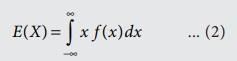Home | | Business Maths 12th Std | Expected value and Variance

# Expected value and Variance

The expected value is a weighted average of the values of a random variable may assume. The weights are the probabilities.

Expected value and Variance

## Expected value

The expected value is a weighted average of the values of a random variable may assume. The weights are the probabilities.

## Definition 6.8

Let X be a discrete random variable with probability mass function (p.m.f.) p(x) . Then, its expected value is defined byIf X is a continuous random variable and f(x) is the value of its probability density function at x, the expected value of X isNote

In (1), E(X) is defined to be the indicated series provided that the series is absolutely convergent; otherwise, we say that the mean does not exist.

In (1), E(X) is an “average” of the values that the random variable takes on, where each value is weighted by the probability that the random variable is equal to that value. Values that are more probable receive more weight.

In (2), E(X) is defined to be the indicated integral if the integral exists; otherwise, we say that the mean does not exist.

In (2), E(X) is an “average” of the values that the random variable takes on, where each value x is multiplied by the approximate probability that X equals the value x, namely f X (x)dx and then integrated over all values.

## Variance

The variance is a weighted average of the squared deviations of a random variable from its mean. The weights are the probabilities. The mean of a random variable X, defined in (1) and (2), was a measure of central location of the density of X. The variance of a random variable X will be a measure of the spread or dispersion of the density of X or simply the variability in the values of a random variable.

## Definition 6.9

The variance of X is defined byif X is discrete random variable with probability mass function p(x).if X is continuous random variable with probability density function f X (x) .

## Definition 6.10

Expected value of [XE(X)]2 is called the variance of the random variable.Note

·        In the following examples, variance will be found using definition 6.10.

·        The variances are defined only if the series in (3) is convergent or if the integrals in (4) exist.

·        If X is a random variable, the standard deviation of X (S.D(X)), denoted by σX , is defined as·        The variance of X , denoted by σX2  or Var(X) or V(X)

Tags : Definition, Formulas | Mathematical Expectation , 12th Business Maths and Statistics : Chapter 6 : Random Variable and Mathematical Expectation
Study Material, Lecturing Notes, Assignment, Reference, Wiki description explanation, brief detail
12th Business Maths and Statistics : Chapter 6 : Random Variable and Mathematical Expectation : Expected value and Variance | Definition, Formulas | Mathematical Expectation